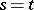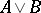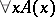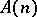# Realizability

(diff) ← Older revision | Latest revision (diff) | Newer revision → (diff)

One of the forms of non-classical interpretation of logical and logico-mathematical languages. Various interpretations of realizability type are defined by the following scheme. For formulas of a logico-mathematical language one defines the relation "an object e realizes a closed formula F" , written "e r F" , for short. The definition is of an inductive nature: The relationis first defined for atomic formulas, and then for compound formulas under the assumption that the relation is already defined for the subformulas from which they are made up. A closed formulais called realizable, or true for a given interpretation, if there is an objectsuch that. A formula, containing free variables, is assumed to be realizable if the closed formulais realizable.

Such an interpretation, known as recursive realizability, was first proposed by S.C. Kleene (see , ), with the aim of giving an intuitionistic (constructive) semantics of the language of formal arithmetic in terms of recursive functions (cf. Recursive function). Other notions of realizability are modifications of recursive realizability.

The intuitive sense of the relationis as follows: The objectencodes information from which one can derive the truth of the formula. For example, in recursive realizability, the natural number 0 realizes an elementary formula of the formif and only if this formula is true (i.e. the values of the termsandcoincide); if a numberrealizes the disjunction, thenencodes which of the formulasoris realizable, and produces a number realizing this formula. If a numberrealizes the formula, thenencodes an algorithm which, for any natural number, produces a realization of the formula. As realizers, i.e. objects which realize formulas, one can almost always take natural numbers. However, in the intuitionistic interpretation of the language of mathematical analysis, other objects can be used as realizers, such as unary number-theoretical functions (see, for example, ).

For the formulas of a logical language, such as propositional or predicate formulas, realizability is usually defined using the concept of realizability for some logico-mathematical language. A logical formulais said to be realizable if every formula in the languagethat can be obtained fromby substituting formulas offor the predicate variables is realizable.

Realizability interpretations have found wide application in the study of non-classical, mostly intuitionistic and constructive, logical and logico-mathematical theories. For a description of various notions of realizability and their applications in proof theory for investigations in intuitionistic theories see , .

How to Cite This Entry:
Realizability. Encyclopedia of Mathematics. URL: http://encyclopediaofmath.org/index.php?title=Realizability&oldid=18553
This article was adapted from an original article by V.E. Plisko (originator), which appeared in Encyclopedia of Mathematics - ISBN 1402006098. See original article